## EX 7.6 QUESTION 1.

1. Solve

(a) 2 / 3 + 1 / 7

(b) 3 / 10 + 7 / 15

(c) 4 / 9 + 2 / 7

(d) 5 / 7 + 1 / 3

(e) 2 / 5 + 1 / 6

(f) 4 / 5 + 2 / 3

(g) 3 / 4 – 1 / 3

(h) 5 / 6 – 1 / 3

(i) 2 / 3 + 3 / 4 + 1 / 2

(j) 1/ 2 + 1 / 3 + 1 / 6

(k)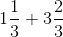(l)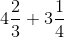(m) 16 / 5 – 7 / 5

(n) 4 / 3 – 1 / 2

Solution:

(a) 2 / 3 + 1/ 7

Taking LCM

[(2 × 7) + (1 × 3)] / 21

= (14 + 3) / 21

= 17 / 21

(b) 3 / 10 + 7 / 15

Taking LCM 30

= [(3 × 3) + (7 × 2)] / 30

= (9 + 14) / 30

= 23 / 30

(c) 4 / 9 + 2/ 7

Taking LCM 63

= [(4 × 7) + (2 × 9)] / 63

= (28 + 18) / 63

= 46 / 63

(d) 5 / 7 + 1 / 3

Taking LCM 21

= [(5 × 3) + (1 × 7)] / 21

= (15 + 7) / 21

= 22 / 21

(e) 2 / 5 + 1 / 6

Taking LCM 30

= [(2 × 6) + (1 × 5)] / 30

= (12 + 5) / 30

= 17 / 30

(f) 4 / 5 + 2 / 3

Taking LCM 15

= [(4 × 3) + (2 × 5)] / 15

= (12 + 10) / 15

= 22 / 15

(g) 3 / 4 – 1 / 3

Taking LCM 12

= [(3 × 3) – (1 × 4)] / 12

= (9 – 4) / 12

= 5 / 12

(h) 5 / 6 – 1 / 3

Taking LCM 6

= [(5 × 1) – (1 × 2)] / 6

= (5 – 2) / 6

= 3 / 6

= 1 / 2

(i) 2 / 3 + 3 / 4 + 1 / 2

Taking LCM 12

= [(2 × 4) + (3 × 3) + (1 × 6)] / 12

= (8 + 9 + 6) / 12

= 23 / 12

(j) 1 / 2 + 1 / 3 + 1 / 6

Taking LCM 6

= [(1 × 3) + (1 × 2) + (1 × 1)] / 6

= (3 + 2 + 1) / 6

= 6 / 6

= 1

(k)= [(3 × 1) + 1] / 3 + [(3 × 3) + 2] / 3

= (3 + 1) / 3 + (9 + 2) / 3

= 4/ / 3 + 11 / 3

= (4 + 11) / 3

= 15 / 3

= 5

(l)= [(3 × 4) + 2] / 3 + [(3 × 4) + 1] / 4

= 14 / 3 + 13 / 4

= [(14 × 4) + (13 × 3)] / 12

= (56 + 39) / 12

= 95 / 12

(m) 16 / 5 – 7 / 5

= (16 – 7) / 5

= 9 / 5

(n) 4 /3 – 1 / 2

Taking LCM 6

= [(4 × 2) – (1 × 3)] / 6

= (8 – 3) /6

= 5 / 6

## EX 7.6 QUESTION 2.

Sarita bought 2 / 5 metre of ribbon and Lalita 3 /4 metre of ribbon. What is the total length of the ribbon they bought?

Solution:

The length of ribbon bought by Sarita = 2 / 5 metre

The length of ribbon bought by Lalita = 3 / 4 metre

Total length of the ribbon bought by both of them = 2 / 5 + 3 / 4

Taking LCM 20

= [(2 × 4) + (3 × 5)] / 20

= (8 + 15) / 20

= 23 / 20 metre

∴ The total length of the ribbon bought by both = 23 / 20 metre

## EX 7.6 QUESTION 3.

Naina was given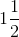piece of cake and Najma was given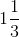piece of cake. Find the total amount of cake was given to both of them.

Solutions:

Fraction of cake Naina got == 3 / 2

Fraction of cake Najma got == 4 / 3

Total amount of cake given to both of them = 3 / 2 + 4 / 3

= [(3 × 3) + (4 × 2)] / 6

= (9 + 8) / 6

= 17 / 6

=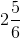## EX 7.6 QUESTION 4.

Fill in the boxes:

(a) ▯ – 5 / 8 = 1 / 4

(b) ▯ – 1 / 5 = 1 / 2

(c) 1 / 2 – ▯ = 1 / 6

Solutions:

(a) ▯ – 5 / 8 = 1 / 4

▯ = 1 / 4 + 5 / 8

▯ = [(1 × 2 + 5)] / 8

▯ = 7 / 8

(b) ▯ – 1 / 5 = 1 / 2

▯ = 1 / 2 + 1 / 5

▯ = [(1 × 5) + (1 × 2)] / 10

▯ = (5 + 2) / 10

▯ = 7 / 10

(c) 1 / 2 – ▯ = 1 / 6

▯ = 1 / 2 – 1 / 6

▯ = [(1 × 3) – (1 × 1)] / 6

▯ = (3 – 1) / 6

▯ = 2 / 6

▯ 1 / 3

## EX 7.6 QUESTION 5.

5. Complete the addition and subtraction box.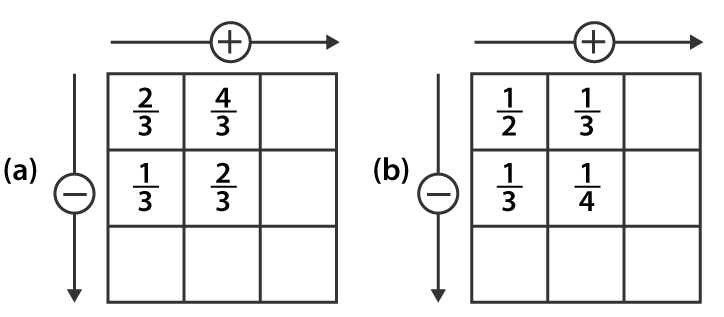Solution:

(a) 2 / 3 + 4 / 3

= (2 + 4) / 3

= 6 / 3

= 2

1 / 3 + 2 / 3

= (1 + 2) / 3

= 3 / 3

= 1

2 / 3 – 1 / 3

= (2 – 1) / 3

= 1 / 3

4 / 3 – 2 / 3

= (4 – 2) / 3

= 2 / 3

1 / 3 + 2 / 3

= (1 + 2) / 3

= 3 / 3

= 1

Hence, the complete given box is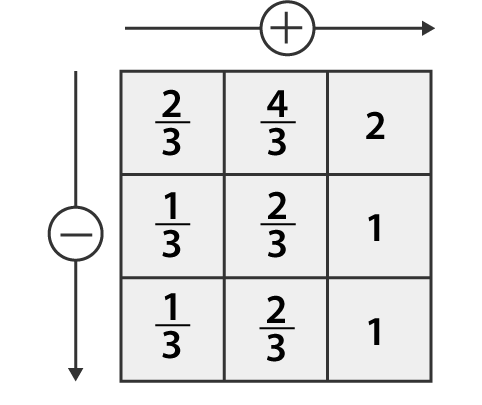(b) 1 / 2 + 1 / 3

= [(1 × 3) + (1 × 2)] / 6

= (3 + 2) / 6

= 5 / 6

1 / 3 + 1 / 4

= [(1 × 4) + (1 × 3)] / 12

= (4 + 3) / 12

= 7 / 12

1 / 2 – 1 / 3

= [(1 × 3) – (1 × 2)] / 6

= (3 – 2) / 6

= 1 / 6

1 / 3 – 1 / 4

= [(1 × 4) – (1 ×3)] / 12

= (4 – 3) / 12

= 1 / 12

1 / 6 + 1 / 12

= [(1 × 2) + 1] / 12

= (2 + 1) / 12

= 3 / 12

= 1 / 4

Hence, the complete given box is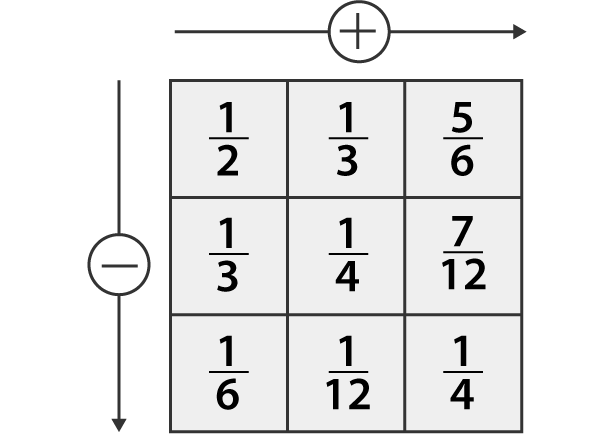## EX 7.6 QUESTION 6.

A piece of wire 7 / 8 metre long broke into two pieces. One-piece was 1 / 4 metre long. How long is the other piece?

Solutions:

Total length of wire = 7 / 8 metre

Length of one piece of wire = 1 / 4 metre

Length of other pieces of wire = Total length of wire –  one piece of wire

= 7 / 8 – 1 / 4

= [(7 × 1) – (1 × 2)] / 8

= (7 – 2) / 8

= 5 / 8

∴ Length of the other piece of wire = 5 / 8 metre

## EX 7.6 QUESTION 7.

Nandini’s house is 9 / 10 km from her school. She walked some distance and then took a bus for 1 / 2 km to reach the school. How far did she walk?

Solutions:

The distance of the school from house = 9 / 10 km

The distance she travelled by bus = 1 / 2 km

Distance walked by Nandini = Total distance of the school – Distance she travelled by bus

= 9 / 10 – 1 / 2

= [(9 × 1) – (1 × 5)] / 10

= (9 – 5) / 10

= 4 / 10

= 2 / 5 km

∴ Distance walked by Nandini is 2 / 5 km

## EX 7.6 QUESTION 8.

Asha and Samuel have bookshelves of the same size partly filled with books. Asha’s shelf is 5 / 6 th full and Samuel’s shelf is 2/ 5 th full. Whose bookshelf is more full? By what fraction?

Solution:

Fraction of Asha’s bookshelf = 5 / 6

Fraction of Samuel’s bookshelf = 2 / 5

Convert these fractions into like fractions

5 / 6 = 5 / 6 × 5 / 5

= (5 × 5) / (6 × 5)

= 25 / 30

2 / 5 = 2 / 5 × 6 / 6

= (2 × 6) / (5 × 6)

= 12 / 30

25 / 30 > 12 / 30

5 / 6 > 2 / 5

∴ Asha’s bookshelf is more full than Samuel’s bookshelf

Difference = 5 / 6 – 2 / 5

= 25 / 30 – 12 / 30

= 13 / 30

## EX 7.6 QUESTION 9.

Jaidev takes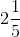minutes to walk across the school ground. Rahul takes 7 / 4 minutes to do the same. Who takes less time and by what fraction?

Solution:

Time taken by Jaidev to walk across the school ground == 11 / 5 minutes

Time taken by Rahul to walk across the school ground = 7 / 4 minutes

Convert these fractions into like fractions

11 / 5 = 11 / 5 × 4 / 4

= (11 × 4) / (5 × 4)

= 44 / 20

7 / 4 = 7 / 4 × 5 / 5

= (7 × 5) / (4 × 5)

= 35 / 20

Clearly, 44 / 20 > 35 / 20

11 / 5 > 7 / 4

∴ Rahul takes less time than Jaidev to walk across the school ground

Difference = 11 / 5 – 7 / 4

= 44 / 20 – 35 / 20

= 9 / 20

Hence, Rahul walks across the school ground by 9 / 20 minutes

error: Content is protected !!